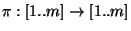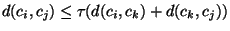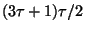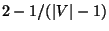Next: MINIMUM GEOMETRIC TRAVELING SALESPERSON Up: Routing Problems Previous: MINIMUM TRAVELING SALESPERSON   Index

### MINIMUM METRIC TRAVELING SALESPERSON PROBLEM

• INSTANCE: Set C of m cities, distancessatisfying the triangle inequality.

• SOLUTION: A tour of C, i.e., a permutation.

• MEASURE: The length of the tour.

• Good News: Approximable within 3/2 .

• Comment: Transformation from MAXIMUM 3-SATISFIABILITY. Variation in which the distances are only 1 and 2 is approximable within 7/6  but not within 5381/5380for any. If the distance function is asymmetric the problem is approximable within. The asymmetric problem with distances 1 and 2 is approximable within 17/12  but not within 2805/2804for any. Variation in which the distances are only 1, 2 or 3 is not approximable within 3813/3812for any(transformation from 3-OCCURRENCE E2-LIN-2 lower bound ). The special case in which the distances are the shortest path lengths in a given weighted planar graph admits a PTAS . Does not admit a PTAS for dense instances even if restricted to distances 1 and 2 .
Variation in which the triangle inequality is relaxed toforis approximable within.
The generalization in which, for each city, a neighborhood is specified in which the salesperson can meet the client, is also approximable for a variety of neighborhood types such as unit segments, unit circles, and unit rectangles . Another generalization in which the salesperson has to rearrange some objects while following the route is approximable within 2.5 . A prize-collecting variation in which a penalty is associated with each vertex and the goal is to minimize the cost of the tour and the vertices not in the tour is approximable within. A clustered generalization in which vertices are partitioned into clusters that must be traversed consecutively is approximable within 2.75 in the general case, within 2.643 if the starting vertex in each cluster is given, within 1.9091 if the starting and ending vertices of each cluster are specified, within 1.8 if in each cluster two vertices are given and we are free to choose any one as starting vertex and the other as ending vertex . A variation in which vertices can be revisited and the goal is to minimize the sum of the latencies of all vertices, where the latency of a vertex c is the length of the tour from the starting point to c, is approximable within 29 and is APX-complete . A combination of this problem and the matching problem, also called Printed Circuit Board Assembly, is approximable within 2.5 . Finally, the variation in which a Hamiltonian path is looked for rather than a tour is also approximable within 1.5 in the general case while if both end vertices are specified it is approximable within 5/3 .